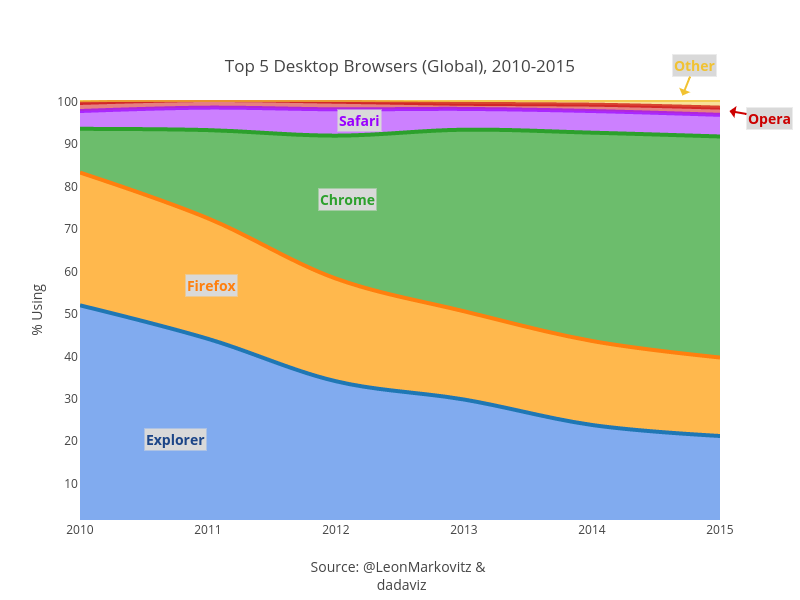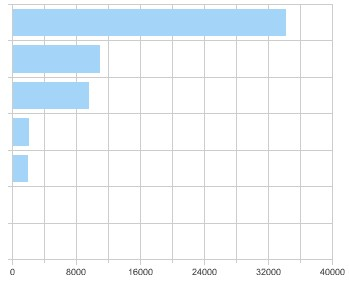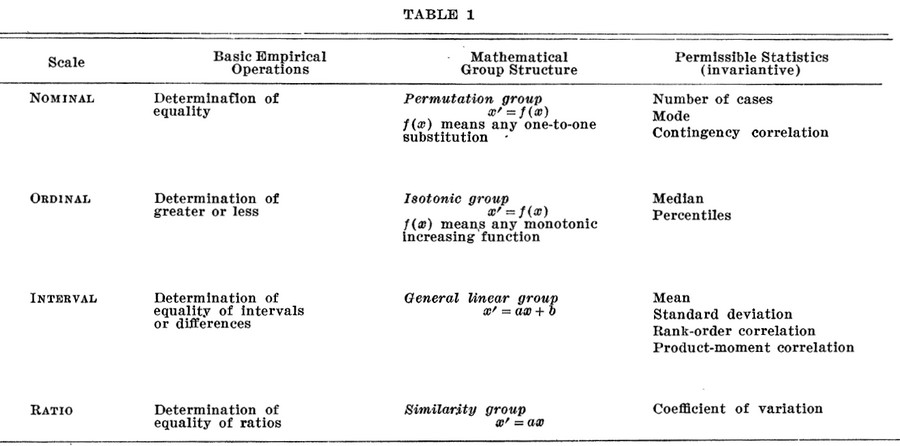# Statistics - (Fraction|Ratio|Percentage|Share) (Variable|Measurement)

Ratio measurement gets its name from the fact that meaningful fraction (ratio or rational number) can be constructed with a ratio variable.

Ratio is the deepest, most meaningful level of measurement, and consequently, the most useful.

A variable measured at this level not only includes the concepts of order and distance, but also adds the idea of “nothingness”, absolute zero or True Zero.

Because of this absolute zero value (“true zero”), the ratio between any two values has a meaning

In the Celsius temperature scale the temperature at which water freezes is really an arbitrarily chosen number and does not represent the absence of temperature. As a result, the ratio between temperatures is relative.

## Meaningless

A ratio must have meaning. A ratio between two nominal variables is meaningless (i.e. a person who scores A did not necessarily do “twice as well” as a student who scored B )

## Type

Two type of ratio measure:

• Percentage measure
• Share measure

## Example

In each of these cases there is an absolute zero that has real meaning

• Height,
• Weight,
• Population (Population = 0 = extinct)
• Age (Age = 0 literally means NO age)
• Temperature K° (the Kelvin scale)

Recommended PagesContrast (Light vs Dark Color Ratio)

Color - Contrast (Light vs Dark Color Ratio) contrast is a luminance ratio showing the difference between the most lighter color and the most darker color of an object. Generally, the contrast is calculated...Data Visualisation - Area Chart

An area chart shows the trend of the contribution of: each value over time or by category. It is basically a line graph for which the regions between lines are filled in. Regions stack, adding...Data Visualization - Bar Chart

Bar graphs plots: a categorical variable associated with quantities (number of case, sum of) histograms continuous variable associated with its bins They show: quantities as bar lengths...Dimensional Data Modeling - Measure

In a dimensional model, a measure is a quantitative attribute of a fact (in a fact table) that is not a foreign key that creates a relationship to a dimension. A measure permits to quantify. A calculated...Logical Data Modeling - (Derived|Calculated) Attribute (Derived Data)

Derived is a property of: a variable (scalar) or a of column (collection) The value can be calculated or deduced from relationship Groupings or from the values of other variable. The value of a Derived...
Number System - Rational Number -

A rational number is any number that can be expressed as the quotient or fraction p/q of two integers Rational numbers are also known as: the rationals, the field of rationals or the field of...Statistics - (Scales of measurement|Type of variables)

According to Stevens theory (On the Theory of Scales of Measurement), the four scales of measurement are: nominal ordinal ...